login  home  contents  what's new  discussion  bug reports help  links  subscribe  changes  refresh  edit

# Edit detail for #191 exquo and therefore gcd cannot handle UP(x, EXPR INT) revision 6 of 6

 1 2 3 4 5 6 Editor: test1 Time: 2017/02/23 19:30:42 GMT+0 Note:

added:

From test1 Thu Feb 23 19:30:42 +0000 2017
From: test1
Date: Thu, 23 Feb 2017 19:30:42 +0000
Subject:
Message-ID: <20170223193042+0000@axiom-wiki.newsynthesis.org>

Status: open => closed



 Submitted by : (unknown) at: 2007-11-17T22:05:44-08:00 (16 years ago) Name : Axiom Version : default friCAS-20090114 Axiom-20050901 OpenAxiom-20091012 OpenAxiom-20110220 OpenAxiom-Release-141 Category : Axiom Aldor Interface Axiom Compiler Axiom Library Axiom Interpreter Axiom Documentation Axiom User Interface building Axiom from source lisp system MathAction Doyen CD Reduce Axiom on Windows Axiom on Linux Severity : critical serious normal minor wishlist Status : open closed rejected not reproducible fix proposed fixed somewhere duplicate need more info Optional subject :   Optional comment :

(new) exquo and therefore gcd cannot handle UP(x, EXPR INT) --Bill Page, Mon, 11 Jul 2005 15:56:25 -0500 reply
Update of bug #10530 (project axiom):

Status: None => transferred

fricas
(1) -> gcd((x-2^a)::UP(x, EXPR INT), simplify((x-2^a)*(x+2^a))::UP(x, EXPR INT))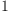(1)
Type: UnivariatePolynomial(x,Expression(Integer))

Gives 1, while the correct answer should be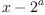, as given by

fricas
gcd((x-2^a)::UP(x, EXPR INT),((x-2^a)*(x+2^a))::UP(x, EXPR INT))(2)
Type: UnivariatePolynomial(x,Expression(Integer))

In fact, to ensure that algebraic relations are respected inside expressions one has to normalize all involved expressions:

fricas
uP := UP(x, EXPR INT)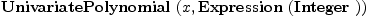(3)
Type: Type
fricas
p1 := (x-2^a)::uP(4)
Type: UnivariatePolynomial(x,Expression(Integer))
fricas
p2 := simplify((x-2^a)*(x+2^a))::uP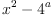(5)
Type: UnivariatePolynomial(x,Expression(Integer))
fricas
cl1 := coefficients(p1)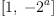(6)
Type: List(Expression(Integer))
fricas
cl2 := coefficients(p2)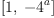(7)
Type: List(Expression(Integer))
fricas
ncl := normalize(concat(cl1, cl2))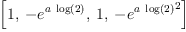(8)
Type: List(Expression(Integer))
fricas
ncl1 := first(ncl, #cl1)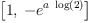(9)
Type: List(Expression(Integer))
fricas
ncl2 := rest(ncl, #cl1)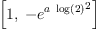(10)
Type: List(Expression(Integer))
fricas
np1 := reduce(_+, [monomial(c,degree(m))$uP for m in monomials(p1) for c in ncl1])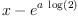(11) Type: UnivariatePolynomial(x,Expression(Integer)) fricas np2 := reduce(_+, [monomial(c,degree(m))$uP for m in monomials(p2) for c in ncl2])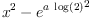(12)
Type: UnivariatePolynomial(x,Expression(Integer))
fricas
gcd(np1, np2)(13)
Type: UnivariatePolynomial(x,Expression(Integer))

Not nice, but in general this is what is needed (below are simpler attempts which sometimes work, but may fail).

Internal Cause

In EXPR INT,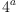and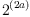are treated as two variables without relations in EXPR INT. But

fricas
simplify((x-2^a)*(x+2^a))::UP(x, EXPR INT)(14)
Type: UnivariatePolynomial(x,Expression(Integer))

Therefore exquo in:

  exquo(simplify((x-2^a)*(x+2^a))::UP(x,EXPR INT),(x-2^a)::UP(x,EXPR INT))


fails. In details, it calls exquo$UP(x,EXPR INT). This implements exact division of polynomials p1 by p2 as usual. After each subtraction - done via fmecg$UP - the result is again stored in p1. exquo terminates when p1 is the empty list - note that UPs? are stored as lists of pairs (degree, coefficient) - or the degree of p2 is larger than p1. In the latter case, exquo fails.

Thus, in our case, at one point p1 is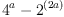, which is zero mathematically, but FriCAS does not know it. In particular, p1 is not the empty list, but rather a constant polynomial...

To get correct result we need to express coefficients of p1 and p2 in terms of independent kernels. In more general contexts (in particular involving abs) this is undecidable, but for elementary functions normalize works. More precisely, currently normalize ignores possible dependencies between algebraic quantities like roots. And to simplification of transcendental constants depends on Schanuel conjecture (if Schanuel conjecture is false we may miss some dependencies).

## Wed 09/29/2004 at 16:02, comment #1:

fricas
exquo(normalize(simplify(((A-2^a)*(A+2^a)))::EXPR INT),normalize((A-2^a)::EXPR INT))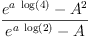(15)
Type: Union(Expression(Integer),...)
fricas
exquo(simplify((A-2^a)*(A+2^a))::UP(A,EXPR INT),(A-2^a)::UP(A,EXPR INT))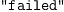(16)
Type: Union("failed",...)

This shows that using normalize separately on polynomials does not help, we need to normalize list of coefficients as a whole.

Few examples showing that without normalization FriCAS does not always treat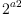the same as:

fricas
dom:=UP('x,EXPR INT)(17)
Type: Type
fricas
p:dom:=x-2^a(18)
Type: UnivariatePolynomial(x,Expression(Integer))
fricas
q:=(x-2^a)*(x+2^a)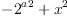(19)
Type: Expression(Integer)
fricas
qq:= simplify(q)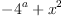(20)
Type: Expression(Integer)
fricas
r:= q::dom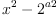(21)
Type: UnivariatePolynomial(x,Expression(Integer))
fricas
rr:= qq::dom(22)
Type: UnivariatePolynomial(x,Expression(Integer))
fricas
gcd(p,q)(23)
Type: UnivariatePolynomial(x,Expression(Integer))
fricas
exquo(q,p)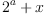(24)
Type: Union(Expression(Integer),...)
fricas
gcd(p,r)(25)
Type: UnivariatePolynomial(x,Expression(Integer))
fricas
gcd(p,qq)(26)
Type: UnivariatePolynomial(x,Expression(Integer))
fricas
gcd(p,rr)(27)
Type: UnivariatePolynomial(x,Expression(Integer))
fricas
q - qq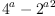(28)
Type: Expression(Integer)
fricas
simplify q - qq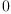(29)
Type: Expression(Integer)
fricas
simplify ((r - rr)::EXPR INT)(30)
Type: Expression(Integer)
fricas
t:Boolean:=(r = rr)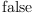(31)
Type: Boolean

Status: open => closed